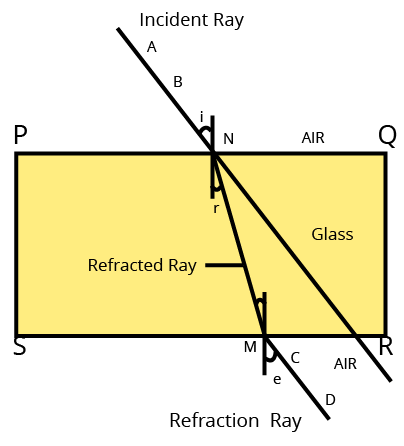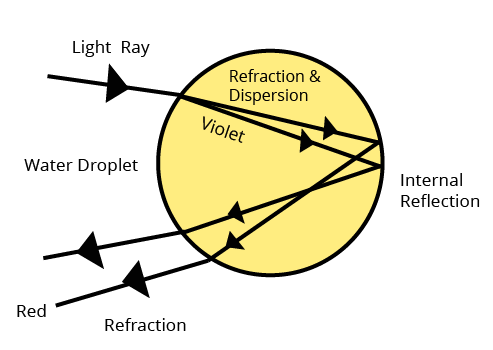Courses
Courses for Kids
Free study material
Free LIVE classes
More

# Maharashtra Board Class 10 Solutions for Science Chapter 6: Refraction of LightLIVE
Join Vedantu’s FREE Mastercalss

## Download Free PDF with Solutions of Refraction of Light Class 10, Chapter 6

In the Maharashtra Board Class 10 syllabus, the chapter Refraction of Light holds a very important place. This chapter discusses some important theories and concepts used behind the Refraction of Light.

The chapter requires a lot of practice, and to understand the chapter properly, students will have to practice from the solutions provided by Vedantu experts. The solutions will cover everything significant about the chapter so that students don’t have to miss out on important details about the sub-topics and problems of the chapter. For further help in solving questions from the chapter, download Free PDF with Solutions of Class 10 Chapter 6 Refraction of Light .

Last updated date: 18th Sep 2023
Total views: 93.9k
Views today: 1.93k

## Access Maharashtra Board Solutions for Class 10 Science Chapter 6 Refraction of Light

1. Fill in the blanks and explain the completed sentences.

1. The Refractive index depends on the ............. of light.

2. The change in ................ of light rays while going from one medium to another is called refraction.

Ans:

a. Wavelength.

Refraction is the term used to describe the simple bending of light caused by the change in speed. The refraction will be greater the more the wavelength changes, hence the medium's refractive index will directly rely on the wavelength.

b. Direction of propagation

The ray of light is believed to move obliquely from one medium to another.

2. Prove the following statements.

1. If the angle of incidence and angle of emergence of a light ray falling on a glass slab are i and e respectively, prove that, i = e.

2. A rainbow is the combined effect of the refraction, dispersion, and total internal reflection of light.

Ans:

a) In the following figure, SR || PQ and NM is the refracted ray

Hence, r = i1.
Now gna = sin i/sin r and ang = sin i1/ sin e.Also gna = $1$/ gna

Sin i /Sin r = Sin i / Sin i =1

As r = i

It follows that Sin i = Sin r

Where gna = refractive index of glass and air.

i = incidence angle

r = reflection angle

b)

1. The refraction, dispersion, internal reflection and further refraction of sunlight by water droplets present in the atmosphere form a rainbow in the sky.2. There are seven colours in the sunlight, including violet, indigo, blue, green, yellow, orange and red. The atmosphere is full of water droplets after it has stopped raining. When sunlight strikes a water droplet, three things happen

• light is refracted and dispersed as it travels from air to water.

• light is internally reflected.

• light is refracted as it travels from water to air.

3. Various colours have different refractive index with violet having the highest and red having the lowest. As a result light disperses (separates into distinct colours) as it travels from air to water.

4. A rainbow with red on the outside and violet on the inside is created by the combined action of various water droplets acting as small prisms. Between these two are the other five colours.

When the sun is behind the viewer and there are water droplets in front than a rainbow can be seen.

3. Mark the correct answer in the following questions.

A. What is the reason for the twinkling of stars?

1. Explosions occurring in stars from time to time

2. Absorption of light in the earth’s atmosphere

3. Motion of stars

4. Changing refractive index of the atmospheric gases.

Ans: The correct option is (iv) Changing refractive index of the atmospheric gases.

Because of atmospheric refraction, stars twinkle.  The passage of light through many layers is what causes stars to twinkle. The densities and temperatures of these layers vary. This caused light beams to bend (refract) several times in a random direction.dg

B. We can see the Sun even when it is little below the horizon because of

1. Reflection of light

2. Refraction of light

3. Dispersion of light

4. Absorption of light

Ans: The correct option is (ii) Refraction of light.

Due to light, we can see the Sun even when it is slightly below the horizon.

Before they enter the Earth's atmosphere, the Sun's light beams travel in a straight path. The Sun's light beams obliquely enter the Earth's atmosphere. Refraction causes the light rays from the Sun to bend and this bending gets more severe as the refractive index of the successive layers gets thicker and thicker. We see the Sun early as a result of the light rays being bent by this. The same effect of light rays bending also causes us to see the Sun's apparent position at sunset rather than its real position.

C. If the refractive index of glass with respect to air is ${\bf{3}}/{\bf{2}}$, what is the refractive index of air with respect to glass?

1. $1/{\bf{2}}$

2. ${\bf{3}}$

3. $1/3$

4. $2/3$

Ans: The correct option is (iv) 2/3

$\begin{array}{l}^a{\mu _g} = \dfrac{{{n_a}}}{{{n_g}}} = \dfrac{3}{2}\\{\;^a}{\mu _g} = \dfrac{{{n_1}}}{{{n_2}}} = \dfrac{2}{3}\end{array}$

4. Solve the following examples:
a) If the speed of light in a medium is${\bf{1}}.{\bf{5}}{\rm{ }} \times {\rm{ }}{\bf{1}}{{\bf{0}}^{\bf{8}}}\;{\bf{m}}/{\bf{s}}$, what is the absolute refractive index of the medium?

Ans: Given

v ( speed of light in a medium$= {\rm{ }}1.5{\rm{ }} \times {\rm{ }}{10^8}\;m/s$

c ( speed of light ) $= {\rm{ }}3{\rm{ }} \times {\rm{ }}{10^8}\;m/s$

n (refractive index of the medium ) = ?

n = cv

$\begin{array}{l} = 3 \times {10^8}m/s\\1.5 \times {10^8}m/s\;\\ = {\rm{ }}2\end{array}$

This is the medium's absolute refractive index.

b. If the absolute refractive indices of glass and water are 3/2 and 4/3 respectively, what is the refractive index of glass with respect to water?

Ans: Given that

ng (refractive index of a glass) $= \dfrac{3}{2}$

nw( refractive index of water) $= \dfrac{4}{3}$

gw(refractive index of glass with respect to water) =?

ng = c / vg

nw c / vw

gw = vw/ vg

gnw= ng / nw

$\begin{array}{l}{n_{\rm{W}}} = \dfrac{c}{{{v_{\rm{W}}}}}\\{{\rm{g}}_{\rm{W}}} = \dfrac{{{v_{\rm{W}}}}}{{{v_{\rm{g}}}}}\\{\rm{g}}{n_{\rm{w}}} = \dfrac{{{n_{\rm{g}}}}}{{{n_{\rm{w}}}}}\\ = \dfrac{{\dfrac{3}{2}}}{{\dfrac{4}{3}}} = \dfrac{{3 \times 3}}{{4 \times 2}}\\ = \dfrac{9}{8}\end{array}$

## Importance of Maharashtra Board textbook solutions for Class 10 Science Refraction of Light

The class 10 Science Chapter 6 Refraction of Light is a crucial chapter included in the syllabus for Maharashtra Board students. The chapter provides information about all the different forms of quadratic equations and how they can be represented. The chapter also talks about the different methods of solving quadratic equations, such as the Factorization Method, the method of completing the square, and the Quadratic Formula. Students will learn about different concepts such as Nature of Roots, Factor Theorem, and Rolle’s Theorem in the chapter.

1. Access to solutions by experts: Getting guidance and learning from experts who have curated the answers that focus on learning standards specific to the age group. It also provides well explained and easy to understand solutions for a student.

2. Beneficial for resolving doubts: Sometimes, questions asked in the chapter become challenging to comprehend, especially for students who find Science difficult. The textbook solutions can present them with elaborative and comprehensive answers or solutions which will benefit them in case of doubts.

3. Learning through mistakes: When a student is trying to solve a question, it is often observed that they get the correct answers but do not follow the proper method. In such cases, textbook solutions can help them understand the experts' approach, which they can use while finding the answers.

4. Practice with the right approach: Practicing the questions by following correct methods and techniques becomes significant for a student. These practice questions become the building blocks for their further question-solving practice. Hence, it is crucial to solving many questions based on a concept with the right approach.

## Benefits of using Vedantus' PDF Solutions for Class 10 Science Maharashtra Board

• No doubt, Refraction of Light is one of the toughest chapters. But the concepts have been cleared with the solutions provided by Vedantu experts. They have explained each and every single process and concept in a very easy language.

• Practising from the Class 10 Science Chapter 6 will help students in building a great foundation for the chapter. The chapter Refraction of Light is a very important chapter in the syllabus, and students will get all the help in preparing for their exams.

• If students have missed out to take notes during their classes, they can easily download the PDF version of the chapter solutions from Vedantu. One can easily access the file, and they can be viewed offline from any mobile device as well.

• Students can use the solutions to revisit any doubts that they have about the chapter and their answers. This can be an aid during the revision for examinations.

## Practice Well With Maharashtra Board Class 10 Science Chapter 6 Solutions

Download Maharashtra board class 10 Science Chapter 6 Refraction of Light and begin practising right now. There are a lot of concepts and formulas that you have to keep in mind for completing the chapter. Hence, early preparation will help you improve your understanding of the chapter. Download the PDF version of these solutions from Vedantu today.